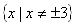Domain

The domain of a rational function is all real numbers, excluding values that lead to a zero in the denominator. These values have been presented as excluded values or points of discontinuity.

Example 1 Describe the Domain.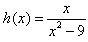Step 1. Factor the denominator.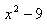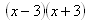Step 2. Using Zero Product Property set each factor equal to zero and solve.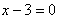or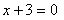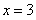or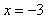Step 3. The domain will be all real numbers excluding the value(s) from step 2.

The domain is: all real numbers excluding x = 3, x = -3.

This can be written in set notation as: